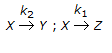# Chemical Engineering - Chemical Reaction Engineering

### Exercise :: Chemical Reaction Engineering - Section 1

31.

The increase in the rate of reaction with temperature is due to

 A. increase in the number of effective collisions. B. decrease in activation energy. C. increase in the average kinetic energy of the reacting molecules. D. none of these.

Explanation:

No answer description available for this question. Let us discuss.

32.

A catalyst loses its activity due to

 A. loss in surface area of the active component. B. agglomeration of metal particles caused by thermal sintering of the solid surface. C. covering of the catalytic active sites by a foregin substance. D. all (a), (b) and (c).

Explanation:

No answer description available for this question. Let us discuss.

33.

'N' plug flow reactors in series with a total volume 'V' gives the same conversion as a single plug flow reactor of volume 'V' for __________ order reactions.

 A. first B. second C. third D. any

Explanation:

No answer description available for this question. Let us discuss.

34.

Specific rate constant for a second order reaction

 A. is independent of temperature. B. varies with temperature. C. depends on the nature of the reactants. D. both (b) and (c).

Explanation:

No answer description available for this question. Let us discuss.

35.

For the irreversible elementary reactions in parallel viz, the rate of disappearance of 'X' is equal to

 A. CA(K1+K2) B. CA(K1 + K2)/2 C. CA . K1/2 D. CA . K2/2